# Olympiad Test: Algebraic Expressions - 1

## 20 Questions MCQ Test Mathematics Olympiad Class 7 | Olympiad Test: Algebraic Expressions - 1

Description
Attempt Olympiad Test: Algebraic Expressions - 1 | 20 questions in 40 minutes | Mock test for Class 7 preparation | Free important questions MCQ to study Mathematics Olympiad Class 7 for Class 7 Exam | Download free PDF with solutions
QUESTION: 1

### Which expression is obtained when 2x2 – 5x + 7 subtracted from 5x2 – 2x + 6?

Solution:

5x2 – 2x + 6 – (2x2 – 5x + 7)
= 5x2 – 2x + 6 – 2x2 + 5x – 7
= 3x2 + 3x – 1

QUESTION: 2

### What is the sum of 5x – 2x2 + 3, 3x2 – 2x + 7 and 4x2 – 5x – 6 ?

Solution:

(5x – 2x2 + 3) + (3x2 – 2x + 7) + (4x2 – 5x – 6)
= (5x – 2x –5x) + (–2x2 + 3x2 + 4x2) + (3 + 7 – 6)
= –2x + 5x2 + 4 = 5x2 – 2x + 4

QUESTION: 3

### What is the result when x2 – xy + y2 subtracted from 2xy – 3y2 + 2x2 ?

Solution:

(2xy – 3y2 + 2x2) – (x2 – xy + y2)
= 2xy – 3y2 + 2x2 – x2 + xy – y2
= x2 + 3xy – 4y2

QUESTION: 4

Find the expression if the sum of 6a – 5a2 + 7 and –7a – 8 + 7a2 is subtracted from –5.

Solution:

–5 – [(6a + 5a2 + 7) + (–7a – 8 + 7a2)]
= –5 – [5a2 +6a + 7 + 7a2– 7a – 8]
= –5 – [12a2 – a – 1] = –5 – 12a2 + a + 1
= –12a2 + a – 4

QUESTION: 5

What is obtained after simplification of the expression [7 – 2x  + 3y –{2x – y}] – (4x + 7y – 5)?

Solution:

[7 – 2x + 3y – {2x – y}] – (4x + 7y – 5)
= 7 – 2x + 3y – 2x + y – 4x – 7y – 5
= –8x – 3y + 12

QUESTION: 6

What is the product of –3m2np, 1/3nm3p2 and 2/3m2n2p2?

Solution: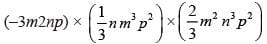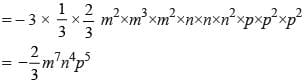QUESTION: 7

What is the product of – xyz2, –2yx2z and 1/3x3yz?

Solution: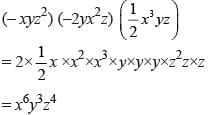QUESTION: 8

5 – (3x + 2y) – 3(x – y) + 7x + y =?

Solution:

5 –(3x + 2y) –3(x – y) + 7x + y
= 5 – 3x – 2y – 3x + 3y + 7x + y
= 5 + x + 2y

QUESTION: 9

Find the product of the sum of 3x2 + 5y2 and x2 – 4y2 and the difference of (x2 – y2) and 2x2 + 3y2.

Solution:

Sum = (3x2 + 5y2) + (x2– 4y2) = 4x2 + y2
Difference = (2x2 + 3y2) –(x2 –y2)
= 2x2 + 3y2 –x2 + y2
= x2 + 4y2
Product = (4x2 + y2) (x2 + 4y2)
= 4x4 + 16x2y2 + x2y2 + 4y4
= 4x4 + 17x2y2 + 4y4

QUESTION: 10

Find the value of (2.3a5b2) × (1.2a2b2) when a = 1 and b = 0.5.

Solution:

(2.3 a5b2) × (1.2a2 – b2)
= 2.3 × 1.2 a7b4
= 2.76 × (1)7 × (0.5)4
= 2.76 × 0.25 × 0.25 = 0.1725

QUESTION: 11

Find the value of (2.6 m2n) × (5mn2) when m = 1/2 and n = 1/3.

Solution:

(2.6 m2n) × (5 mn2)
= 2.6 × 5 × m2× m × n × n2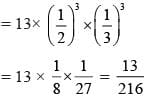QUESTION: 12

Find the product of  ab2c, – a2bc2, –abc3 and –a2bc.

Solution:

(ab2c) (–a2bc2) (–abc3) (–a2bc)
= –a × a2 × a × a2 × b2 × b × b × b × c× c2 × c3 × c
= –a6b5c7

QUESTION: 13

What is obtained when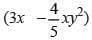is multiplied by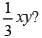Solution: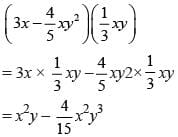QUESTION: 14

Find the numerical value of the product 3s(s2 – st) when s = 2 and t = 5.

Solution:

3s(s2– st)
= 3s3 – 3s2t
= 3(2)3 – 3(2)2×5
= 3×8 – 3×4×5
= 24 – 60 = –36

QUESTION: 15

What is the simplified value of  a(b – c) + b(c – a) + c(a – b)?

Solution:

a(b – c) + b(c – a) + c(a – b)
= ab – ac + bc – ab + ac – bc = 0

QUESTION: 16

Simplify a(b – 2c) + 2b(c – 2a) + c(3a – 2b).

Solution:

a(b – 2c) + 2b(c – 2a) + c(3a – 2b)
= ab – 2ac + 2bc – 4ab + 3ac – 2bc
= ac – 3ab

QUESTION: 17

What is the product of –3x2y2z2 and –5xy2z

Solution:

(–3x2y2z2) (–5xy2z)
= (–3)(–5)x3y4z3
= 15x3y4z3

QUESTION: 18

Find the simplest expression of a(b – c) – b(c – 2a) – c(2a – b)

Solution:

a(b – c) –b(c – 2a) – c(2a – b)
= ab – ac – bc + 2ab – 2ac + bc
= 3ab – 3ac = 3(ab – ac)

QUESTION: 19

What is product of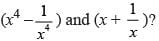Solution: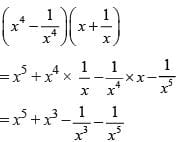QUESTION: 20

What is the product of  (x3 + y3) and (x2 – y2)?

Solution:

(x3 + y3) (x2– y2)
= (x3) (x2) – (x3) (y2) + (y3) (x2) – (y3)(y2)
= x5 – x3y2 + x2y3 – y5Use Code STAYHOME200 and get INR 200 additional OFF Use Coupon Code